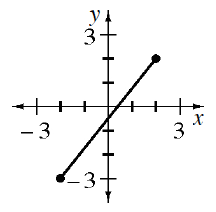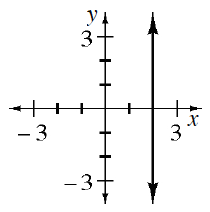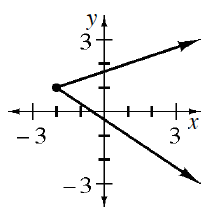Home > CCA > Chapter Ch6 > Lesson 6.1.4 > Problem6-45

6-45.

For each graph below, what are the domain and range? Homework Help ✎

See the Math Notes box in Lesson 1.

1.Domain: $−2\le x\le2$
Range: $−3\le y\le2$

1.Domain: $x=2$
Range: All Numbers

1.1. Which, if any, of the graphs represents a function?

In order for a graph to represent a function, each $x$-value can only relate to one $y$-value.